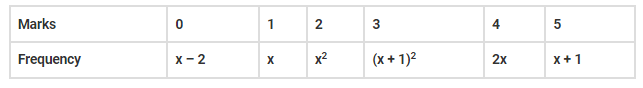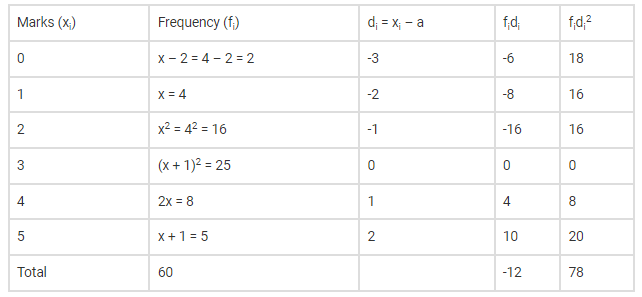# There are 60 students in a class.Question:

There are 60 students in a class. The following is the frequency distribution of the marks obtained by the students in a test:Where x is a positive integer. Determine the mean and standard deviation of the marks.

Solution:

Given there are 60 students in a class. The frequency distribution of the marks obtained by the students in a test is also given.

Now we have to find the mean and standard deviation of the marks.

It is given there are 60 students in the class, so

∑fi=60

⇒ (x – 2) + x + x+ (x + 1)+ 2x + x + 1 = 60

⇒ 5x – 1 + x+ x+ 2x + 1 = 60

⇒ 2x2 +7x = 60

⇒ 2x2 +7x – 60 = 0

Splitting the middle term, we get

⇒ 2x2 + 15x – 8x – 60 = 0

⇒ x (2x + 15) – 4(2x + 15) = 0

⇒ (2x + 15) (x – 4) = 0

⇒ 2x + 15 = 0 or x – 4 = 0

⇒ 2x = -15 or x = 4

Given x is a positive number, so x can take 4 as the only value.

And let assumed mean, a=3.

Now put x = 4 and a = 3 in the frequency distribution table and add other columns after calculations, we getAnd we know standard deviation is

$\sigma=\sqrt{\frac{\sum f_{i} d_{i}^{2}}{n}-\left(\frac{\sum f_{i} d_{i}}{n}\right)^{2}}$

Substituting values from above table, we get

$\sigma=\sqrt{\frac{78}{60}-\left(\frac{-12}{60}\right)^{2}}$

Substituting values from above table, we get

$\sigma=\sqrt{\frac{78}{60}-\left(\frac{-12}{60}\right)^{2}}$

$\sigma=\sqrt{1.3-(0.2)^{2}}$

$\sigma=\sqrt{1.3-0.04}$

$\Rightarrow \sigma=1.12$

Hence the standard deviation is $1.12$

Now mean is

$\overline{\mathrm{x}}=\mathrm{A}+\frac{\sum \mathrm{f}_{\mathrm{i}} \mathrm{d}_{\mathrm{i}}}{\mathrm{N}}$

$=3+\left(-\frac{12}{60}\right)$

$=3-\frac{1}{5}=\frac{14}{5}$

$=2.8$

Hence the mean and standard deviation of the marks are $2.8$ and $1.12$ respectively.